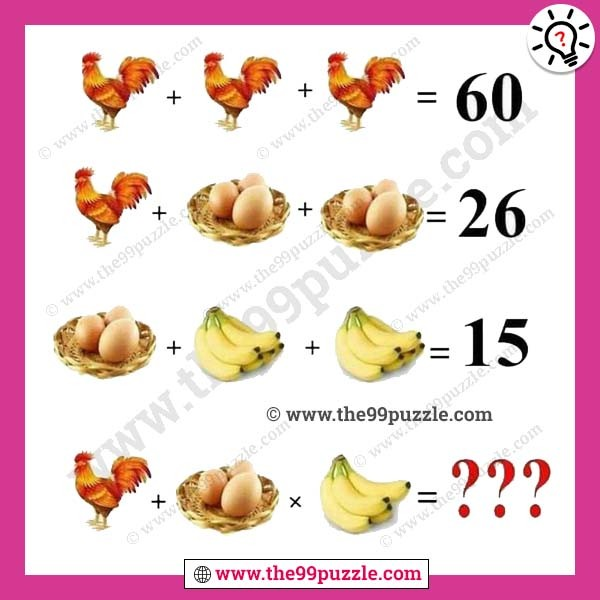# Egg hen bananas puzzle with answer – Puzz113

It is a simple math equation picture puzzle to test your brain. Solve the egg hen bananas puzzle. This is an easy viral math puzzle. However, if you are not clear with the BODMAS rule, you may get a different answer. Let us see if you can answer this math equation picture puzzle correctly and find the value of the missing number which replaces the question mark? Can you find the egg hen bananas puzzle answer?###### Explanation:

Hen = 20

Egg = 1

Banana = 1

Hen + Hen + Hen = 60

Hen + (3×Egg) + (3×Egg)= 26

(3×Egg) + (6×Banana) + (6×Banana)= 15

Hen + (3×Egg) × (5×Banana) = ?

20+20+20=60

20+(3×1)+(3×1)=26

(3×1)+(6×1)+(6×1)=15

20+(3×1)×(5×1)=35